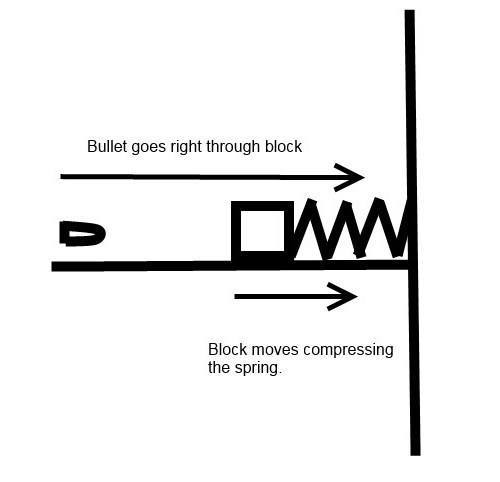# Spring compression after bullet passes through block

## Homework Statement

Its in the screenshot i took. I need someone to walk me through the process of solving this problem because I do not understand how to get the maximum compression distance x of the spring. I know that the momentum of the billet will= the momentum of the block.

## Homework Equations

mgh=-mgx+(1/2)*k*x^2
mg(h+x) = (1/2)* k*x^2

## The Attempt at a Solution

#### Attachments

•Screen Shot 2016-06-14 at 9.53.08 PM.png
22.1 KB · Views: 440
Last edited:

Its in the screenshot i took. I need someone to walk me through the process of solving this problem because I do not understand how to get the maximum compression distance x of the spring. I know that the momentum of the billet will= the momentum of the block.

pl. show us your attempts to solve the problem

pl. show us your attempts to solve the problem
Ok I got
mgh=-mgx+(1/2)*k*x^2
since I don't have a value for h, cause the bullet is traveling horizontal the equation is
mgx=(1/2)*k*x^2
x=((1/2)*k*x^2)/mg
where k=121N/m-this is given
This is as far as I got, cause at this point I am confused, cause if I had h, I would be able to solve this.

billy_joule
Gravitational potential energy is not relevant to the problem at all, there are no changes in mgh for either the bullet, block or spring. So, mgh (or mgx) should not appear in your work anywhere, in fact 'g' should not appear anywhere either.

You first need to find the velocity of the block after the bullet has exited, use conservation of momentum to do this.

I know that the momentum of the billet will= the momentum of the block.

That would only be true if the bullet stops completely, transferring all of it's momentum to the block. We know that's not the case.
What we know is that momentum is always conserved so:
pinitial = pfinal
And so:
pinitial, block + pinitial, bullet = pfinal, block +pfinal, bullet
and of course p =mv, so you have all the information to solve for vblock, final

Once we know the velocity of the block we can find the blocks kinetic energy, then using conservation of energy, solve for the springs compression.

Ok so since the blocks initial velocity is 0 I get the final velocity to be .633 m/s An then for the blocks Kinetic energy I get .18031joules? Are these answers good so far? Also for the conversation of energy the m will be the mass of the block and I assume h will be the springs compression distance?

Last edited:
Sorry for the double post, you can disregard my second question on what m and h are, I was thinking of something else. For the law of conversation of energy what will the equation be? The reason I am asking is because you said I should not have mgh in any of my work, so I'm not sure what to do?

Last edited:
CWatters
Homework Helper
Gold Member
Ok so since the blocks initial velocity is 0 I get the final velocity to be .633 m/s An then for the blocks Kinetic energy I get .18031joules? Are these answers good so far?

Yes. I also get 0.633' and 0.1805 Joules.

Alright cool, I am just puzzled over the conservation of energy which is Ef=Ei, and I don't know what the equation will look like?

I was thinking of sing F=-kx, but then I don't know how to find F? for the block.

Last edited:
CWatters
Homework Helper
Gold Member
ok so the block starts off with some KE. Where does that KE end up?

ok so the block starts off with some KE. Where does that KE end up?
When you say start up you mean the KE that I calculated correct? If so then F=KE, sooo
KE=-k*x
where x=KE/k
Correct?

CWatters
Homework Helper
Gold Member
It ends up in the spring. Look up the equation for the energy stored in a spring.

Edit: Actually I see you mentioned it in your OP.

It ends up in the spring. Look up the equation for the energy stored in a spring.

Edit: Actually I see you mentioned it in your OP.
Yes but I cant use those equations cause the potential energy is 0. You said I can use the kinetic energy and the conservation of energy to find the springs compression x, but I just don't see how I can do it without including mgh, it also makes no sense to use because there is no displacement?

CWatters
Homework Helper
Gold Member
Yes but I cant use those equations cause the potential energy is 0. You said I can use the kinetic energy and the conservation of energy to find the springs compression x, but I just don't see how I can do it without including mgh, it also makes no sense to use because there is no displacement?

Sorry if this is obvious but... You do realise the spring is horizontal not vertical? The situation looks like this drawing...The equation for the KE stored in a spring is 1/2 kx2 where x is the displacement you have been asked to find,

I got it thx. x=.054m

CWatters